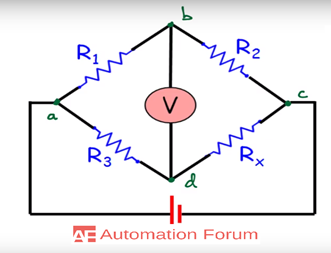# Wheatstone bridge and its applications

What is a Wheatstone bridge

A Wheatstone bridge is used to measure resistance, and they have many other applications like they can be used for calibrating measuring instruments, voltmeters, ammeters. Wheatstone bridge is capable to measure very low resistance values the sensitivity of the Wheatstone bridge is very high so that it can measure small changes in resistance and because of this they can be used in strain gauges. They have the ability to measure with high precision and its precision is much higher when it is compared to a voltmeter, ammeter, and ohmmeters. Wheatstone bridge has many applications, most of the industrial sensors like temperature, humidity, displacement. Shows the value change in resistance. Wheatstone bridge can be used for low resistance measurement so it can provide a very accurate range of measurement by this. So it can be used for many measurement-related applications where minute changes can be determined by using the Wheatstone bridge.

Why Wheatstone bridge is called a bridge

Wheatstone bridge is referred to as a bridge, it is because it is in the form of a bridge. The bridge is useful to measure small changes in resistance. Wheatstone bridge consists of four resistors that are connected in the shape of a diamond.

How does a Wheatstone bridge workWhetstone bridge can be considered as the simple series-parallel arrangement of resistors and they are connected between a supply voltage and ground Wheatstone bridge circuit consists of two input terminals and two output terminals consisting of four resistors. The bridge is used to find the unknown resistance very precisely by comparing it with a known value of resistance. In this circuit, the null or balanced condition is used to find the resistance. For the balanced condition voltage at B and D must be equal which is shown in the above figure so no current will flow through the meter and get a balanced condition one of the resistors must be variable. It’s working procedure is like by using the ratio of three known resistors to measure the fourth unknown resistance, a balanced voltage between the points allows the measurement. It will change the resistors to adjusting variable resistors to balance the device and the mathematical ratio is used to calculate the fourth resistance.

What are the two basic modes of bridge operation

In one mode bridge can measure the unknown resistance value with high accuracy by comparing it with a properly-known resistance, the value of the unknown resistance is measured by varying the resistance of any of the three resistors in the bridge circuit to obtain a balanced condition. In this condition, the bridge will have a zero output voltage. The bridge is in an unbalanced condition in other mode and the unknown resistance value is determined by the value of the bridge output voltage. This mode of operation is also called an off-null operation. The unbalanced bridge is mostly used for dynamic measurement cases in which the unknown resistance will be a transducer which does the measurement of a physical quantity. AC bridge is similar to the Wheatstone bridge and they are used to measure the capacitance and inductance value.

Sensitivity and accuracy of Wheatstone bridge

Wheatstone bridge sensitivity depends upon the change in bridge output voltage due to a small change in resistance of one of the bridge arm, it will be the unknown resistance. The accuracy of the Wheatstone bridge is depended on the accuracy of the other three resistors if their accuracy is good then the accuracy of determining the unknown resistance will be good too.

What are the applications of Wheatstone bridge

Balanced bridge circuits can be useful for many electronics applications such as they can be used to measure the light intensity, pressure, strain. Wheatstone bridge has a wide range of applications for mechanical and electrical applications. Many types of resistive sensors can be used within a Wheatstone bridge circuit they are positional sensors, photo-resistive sensors, temperature sensors.

Wheatstone bridge light detector

Major application of the bridge is the measuring of light with the help of a photo-resistive device. Resistors in the Wheatstone bridge is replaced by a light-dependent resistor. LDR is a resistive sensor that can do the conversion of visible light changes into a resistance change.

Wheatstone bridge for strain measurement

Strain gauges are used in devices where the electrical resistance change by the applied strain and the change in resistance will be a small fraction so in order to measure the small changes in resistance Wheatstone bridges are used

• Wheatstone bridge can measure low resistance values accurately

• Wheatstone bridge can measure physical parameters like temperature, strain, and light with the help of an op-amp

1 Like